login  home  contents  what's new  discussion  bug reports help  links  subscribe  changes  refresh  edit

Try Reduce calculations here. For example:

  \begin{reduce}
solve({z=x*a+2},{z,x});
int(sqrt(1-sin(x)*cos(x)),x);
\end{reduce}


 solve({z=x*a+2},{z,x}); reduce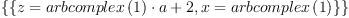int(sqrt(1-sin(x)*cos(x)),x); reduce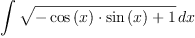example from my daughter's college calc --pbwagner, Mon, 10 Sep 2007 12:58:25 -0500 reply
 int(log(log(x)),x); reduce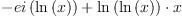fricas
(1) -> integrate(log(log(x)),x)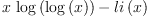(1)
Type: Union(Expression(Integer),...)

 solve({y=-1/l*(1-(l*x+(-3*l^2-l+8)^(1/2)/4-0.5*l)^2)^(1/2) -(-3*l^2-l+8)^(1/2)/(4*l)+2*l}, {y}); reduce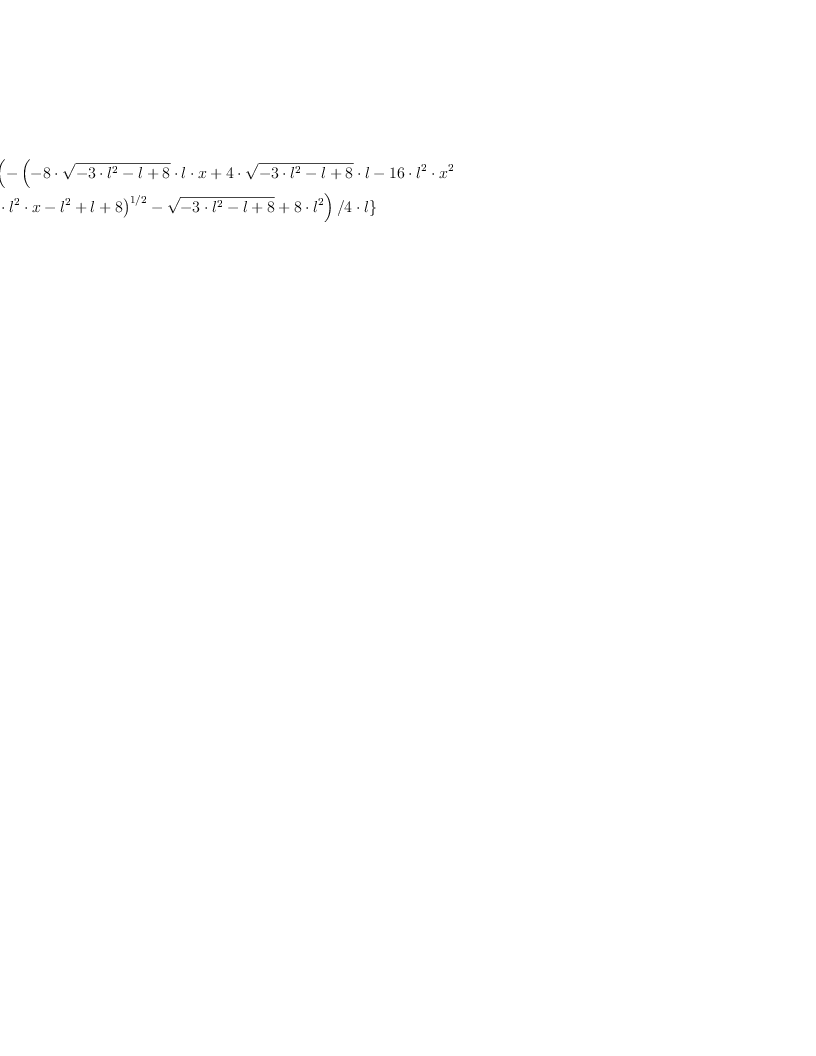how to reduce this to c + atan(tan(d)*(2/x+1)) ?? --haceaton, Fri, 13 Feb 2009 05:47:13 -0800 reply
 trigsimp(atan(((1+x)*sin(c+d)-sin(c-d))/((1+x)*cos(c+d)-cos(c-d))),tan); reduce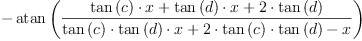compare axiom result --Bill Page, Fri, 13 Feb 2009 07:26:21 -0800 reply
fricas
normalize( atan(((1+x)*sin(c+d)-sin(c-d))/((1+x)*cos(c+d)-cos(c-d))) )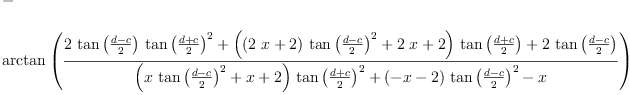(2)
Type: Expression(Integer)

 solve({z=x*a+2},{z,x}); reduce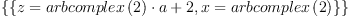int(sqrt(1-sin(x)*cos(x)),x); reducesolve({z=x*a+2},{z,x}); reduce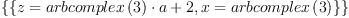int(sqrt(1-sin(x)*cos(x)),x); reduceload_package SPECFN; reduce

 on ROUNDED,ADJPREC; reduce

 Ei(1.00000000000000000001); *** precision increased to 21 reduce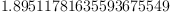Ei(1.0); reduce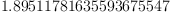Ei(2.0); reduce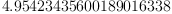Can Reduce compute Ei in arbitrary precision?

Reset

 off ROUNDED,ADJPREC; reduce

 load_package "redlog"; Off TeX; rlset ibalp; *** turned off switch lower Redlog Revision 6174 of 2021-11-15, 15:53:14Z (c) 1992-2021 T. Sturm and A. Dolzmann (www.redlog.eu) type ?; for help {} rlpcvar X1,X2,X3,X22,X4,X10,X23,X5,X6,X7,X14,X13,X20,X12,X21,X11,X18,X17,X16,X15,X9,X8,X19; formooleasli := (X2 and X3) or ((( not X2) and X4) and ( not (X3 or X5))) or (( not (X2 or X4)) and (( not X3) and X5)) and (X2 and X6) or (X2 and ( not (X6 or X7))) or (( not (X2 or X4)) and (( not X6) and X7)) and (X8 and X9 and X6) or ((( not X8) and X10) and ( not (X9 or X11)) and ( not (X6 or X7))) or (( not (X8 or X10)) and (( not X9) and X11) and ( not (X6 or X7))) or (( not (X8 or X10)) and ( not (X9 or X11)) and (( not X6) and X7)) and (X8 and X12) or ((( not X8) and X10) and ( not (X12 or X13))) or (( not (X8 or X10)) and (( not X12) and X13)) and (X14 and X12) or ((( not X14) and X15) and ( not (X12 or X13))) or (( not (X14 or X15)) and (( not X12) and X13)) and (X9 and X16) or ((( not X9) and X11) and ( not (X16 or X17))) or (( not (X9 or X11)) and (( not X16) and X17)) and (X18 and X16) or ((( not X18) and X19) and ( not (X16 or X17))) or (( not (X18 or X19)) and (( not X16) and X17)) and (X18 and X20) or (X18 and (( not X20) and X21)) or (( not (X18 or X19)) and (( not X20) and X21)) and (X22 and X20) or ((( not X22) and X23) and ( not (X20 or X21))) or (( not (X22 or X23)) and (( not X20) and X21)); formooleasli := (x2 = 1 and x3 = 1) or ((not(x2 = 1) and x4 = 1) and not(x3 = 1 or x5 = 1)) or ((not(x2 = 1 or x4 = 1) and not(x3 = 1) and x5 = 1) and x2 = 1 and x6 = 1) or (x2 = 1 and not(x6 = 1 or x7 = 1)) or ( (not(x2 = 1 or x4 = 1) and not(x6 = 1) and x7 = 1) and x8 = 1 and x9 = 1 and x6 = 1) or ((not(x8 = 1) and x10 = 1) and not(x9 = 1 or x11 = 1) and not(x6 = 1 or x7 = 1)) or (not(x8 = 1 or x10 = 1) and (not(x9 = 1) and x11 = 1) and not(x6 = 1 or x7 = 1)) or ( (not(x8 = 1 or x10 = 1) and not(x9 = 1 or x11 = 1) and not(x6 = 1) and x7 = 1) and x8 = 1 and x12 = 1) or ((not(x8 = 1) and x10 = 1) and not(x12 = 1 or x13 = 1)) or ( (not(x8 = 1 or x10 = 1) and not(x12 = 1) and x13 = 1) and x14 = 1 and x12 = 1) or ((not(x14 = 1) and x15 = 1) and not(x12 = 1 or x13 = 1)) or ( (not(x14 = 1 or x15 = 1) and not(x12 = 1) and x13 = 1) and x9 = 1 and x16 = 1) or ((not(x9 = 1) and x11 = 1) and not(x16 = 1 or x17 = 1)) or ( (not(x9 = 1 or x11 = 1) and not(x16 = 1) and x17 = 1) and x18 = 1 and x16 = 1) or ((not(x18 = 1) and x19 = 1) and not(x16 = 1 or x17 = 1)) or ( (not(x18 = 1 or x19 = 1) and not(x16 = 1) and x17 = 1) and x18 = 1 and x20 = 1) or (x18 = 1 and not(x20 = 1) and x21 = 1) or ( (not(x18 = 1 or x19 = 1) and not(x20 = 1) and x21 = 1) and x22 = 1 and x20 = 1) or ((not(x22 = 1) and x23 = 1) and not(x20 = 1 or x21 = 1)) or (not(x22 = 1 or x23 = 1) and not(x20 = 1) and x21 = 1) rlqsat formooleasli; (x10 = 1 and x11 = 0 and x6 = 0 and x7 = 0 and x8 = 0 and x9 = 0) or (x10 = 1 and x12 = 0 and x13 = 0 and x8 = 0) or (x10 = 0 and x11 = 1 and x6 = 0 and x7 = 0 and x8 = 0 and x9 = 0) or (x11 = 1 and x16 = 0 and x17 = 0 and x9 = 0) or (x12 = 0 and x13 = 1 and x14 = 0 and x15 = 0 and x16 = 1 and x9 = 1) or (x12 = 0 and x13 = 0 and x14 = 0 and x15 = 1) or (x16 = 0 and x17 = 0 and x18 = 0 and x19 = 1) or (x18 = 1 and x20 = 0 and x21 = 1) or (x2 = 1 and x3 = 1) or (x2 = 1 and x6 = 0 and x7 = 0) or (x2 = 0 and x3 = 0 and x4 = 1 and x5 = 0) or (x20 = 0 and x21 = 1 and x22 = 0 and x23 = 0) or (x20 = 0 and x21 = 0 and x22 = 0 and x23 = 1) reduce

redlog example --Bill Page, Tue, 06 Mar 2012 16:45:26 -0800 reply
From: http://zengler.eu/research/computer-algebra

 load redlog; Off TeX; *** Off declared operator Off(TeX) rlset ibalp; *** turned on switch lower *** turned off switch lower {ibalp} f := (X or Y or not U) and (not X or not Y or W); f := (x = 1 or y = 1 or not(u = 1)) and (not(x = 1) or not(y = 1) or w = 1) g := ex(x,all(y,all(u,f))); g := ex x all y all u ((x = 1 or y = 1 or not(u = 1)) and (not(x = 1) or not(y = 1) or w = 1)) rlqsat g; w = 1 reduce

test one thing --test int, Sun, 10 Jun 2018 12:54:47 +0000 reply
 int(sqrt(x+sin(x)),x); sqrt(sin(x) + x)*cos(x)*x (2*sqrt(sin(x) + x)*x - int(---------------------------,x) sin(x) + x sqrt(sin(x) + x)*x - int(--------------------,x))/2 sin(x) + x reduce

test another thing ^_^ --test int, Sun, 10 Jun 2018 12:56:14 +0000 reply
 int(sqrt(1-sin(x)*cos(x))*x/(x+sin(x)),x); sqrt( - cos(x)*sin(x) + 1)*x int(------------------------------,x) sin(x) + x reduce

 Subject:   Be Bold !! ( 14 subscribers )